Cylindrical measure

A cylindrical measure in measure theory on topological vector spaces is a finitely-additive measuredefined on the algebra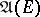of cylinder sets in a topological vector space, that is, sets of the form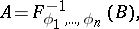(*)

where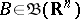— the Borel-algebra of subsets of the space,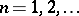;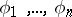are linear functionals on, and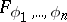is the mapping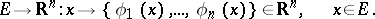Here it is assumed that the restriction ofto any-subalgebra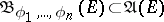of sets of the form (*) with a fixed collection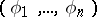of functionals is a-additive measure on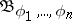(other names are pre-measure, quasi-measure).

In the theory of functions of several real variables a cylindrical measure is a special case of the Hausdorff measure, defined on the Borel-algebra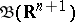of the space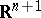by means of the formula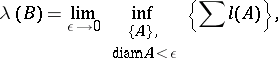where the lower bound is taken over all finite or countable coverings of a set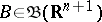by cylinderswith spherical bases and axes parallel to the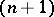-st coordinate axis in; here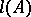is the-dimensional volume of an axial section of the cylinder. Whenis the graph of a continuous functionofvariables defined in a domain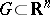:then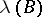is the same as the so-called-dimensional variation of.